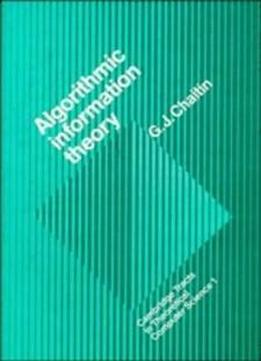# Algorithmic Information Theory (Cambridge Tracts in Theoretical Computer Science) by Gregory. J. Chaitin / 1987 / English / PDF

Chaitin, the inventor of algorithmic information theory, presents in this book the strongest possible version of Gödel's incompleteness theorem, using an information theoretic approach based on the size of computer programs. One half of the book is concerned with studying the halting probability of a universal computer if its program is chosen by tossing a coin. The other half is concerned with encoding the halting probability as an algebraic equation in integers, a so-called exponential diophantine equation.

views: 868